Redlich-Kwong equation of state

The Redlich-Kwong equation of state is :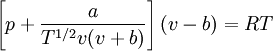$\left[ p + \frac{a}{T^{1/2}v(v+b)} \right] (v-b) = RT$.

The Redlich-Kwong equation of state has a critical point compressibility factor of :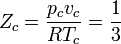$Z_c = \frac{p_c v_c}{RT_c}= \frac{1}{3}$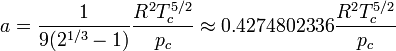$a = \frac{1}{9(2^{1/3}-1)} \frac{R^2T_c^{5/2}}{p_c} \approx 0.4274802336 \frac{R^2T_c^{5/2}}{p_c}$

and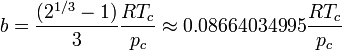$b = \frac{(2^{1/3}-1)}{3} \frac{RT_c}{p_c} \approx 0.08664034995 \frac{RT_c}{p_c}$

where$p$ is the pressure,$T$ is the temperature and$R$ is the molar gas constant.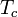$T_c$ is the critical temperature and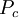$P_c$ is the pressure at the critical point.

Soave Modification

A modification of the the Redlich-Kwong equation of state was presented by Giorgio Soave in order to allow better representation of non-spherical molecules. In order to do this, the square root temperature dependence was replaced with a temperature dependent acentric factor (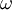$\omega$):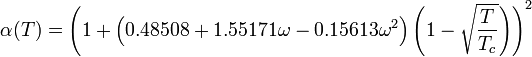$\alpha(T)=\left(1+\left(0.48508+1.55171\omega-0.15613\omega^2\right)\left(1-\sqrt\frac{T}{T_c}\right)\right)^2$

where$T_c$ is the critical temperature. This leads to an equation of state of the form: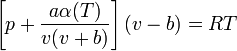$\left[p+\frac{a\alpha(T)}{v(v+b)}\right]\left(v-b\right)=RT$

or equivalently: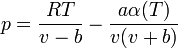$p=\frac{RT}{v-b}-\frac{a\alpha(T)}{v(v+b)}$